﻿ 几种典型的粗糙海面下的声传播计算方法
«上一篇文章快速检索 高级检索

 哈尔滨工程大学学报2019, Vol. 40Issue (4): 781-785,850  DOI: 10.11990/jheu.2017110560

引用本文YAO Meijuan, LU Licheng, GUO Shengming, et al. Several sound propagation calculation methods under rough sea surface[J]. Journal of Harbin Engineering University, 2019, 40(4), 781-785,850. DOI: 10.11990/jheu.201711056.文章历史

1. 中国科学院 声学研究所, 北京 100190;
2. 中国科学院 水声环境特性重点实验室, 北京 100190

Several sound propagation calculation methods under rough sea surface
YAO Meijuan 1,2, LU Licheng 1,2, GUO Shengming 1,2, MA Li 1,2
1. Institute of Acoustics, Chinese Academy of Sciences, Beijing 100190;
2. Key laboratory of underwater Acoustics Environment, Chinese Academy of Sciences, Beijing 100190, China
Abstract: The effect of sea surface roughness on the sound propagation is of great significance to acoustic field predictions in medium-and long-ranges propagation ranges, especially for shallow oceans or oceans with a surface duct.This study investigates several sound propagation calculation methods with surface roughness-Krakenc-Kirchhoff approximation (KA), Krakenc-small slope approximation (SSA), and Ramsurf-to provide a model basis for sound propagation research with sea surface roughness.Moreover, the effectiveness of Ramsurf model was certified by analyzing trial data in high sea state, providing a model for acoustical transmission research under rough surface.It is of great significance to acoustic field prediction and sonar performance prediction.
Keywords: surface roughness    sound propagation    transmission loss    high sea state    surface reflection coefficient    acoustic scattering

Williams等基于PM谱研究了粗糙海面反射系数问题；Jones等[15-17]基于PM谱粗糙海面比较了KA(kirchhoff approximation，KA)散射方法和SSA(small slope approximation，SSA)散射方法计算的海面反射损失，并与海试数据做了比较，还利用Bellhop仿真计算了平滑海面、粗糙海面不考虑气泡影响和粗糙海面考虑气泡影响时的传播曲线。

Ramsurf、Krakenc是已有的粗糙海面传播模型，Krakenc-KA和Krakenc-SSA分别是作者基于KA散射理论和SSA散射理论提出的；本文通过仿真实验比较了几种典型的粗糙海面声传播计算方法，并通过海试数据分析了Ramsurf粗糙海面声传播计算方法的有效性。

1 粗糙海面下的声传播模型 1.1 单粗糙海面下的声传播模型：Krakenc-KA和Krakenc-SSA

 $\mathit{\boldsymbol{r}} = \left( {x, z} \right), {k_i} = ({k_{ix}}, {k_{iz}}) = ({k_{ix}}, -{\kappa _{iz}})$

 ${\kappa _{iz}} = {[{k^2}-{k_{ix}}^2]^{1/2}} > 0$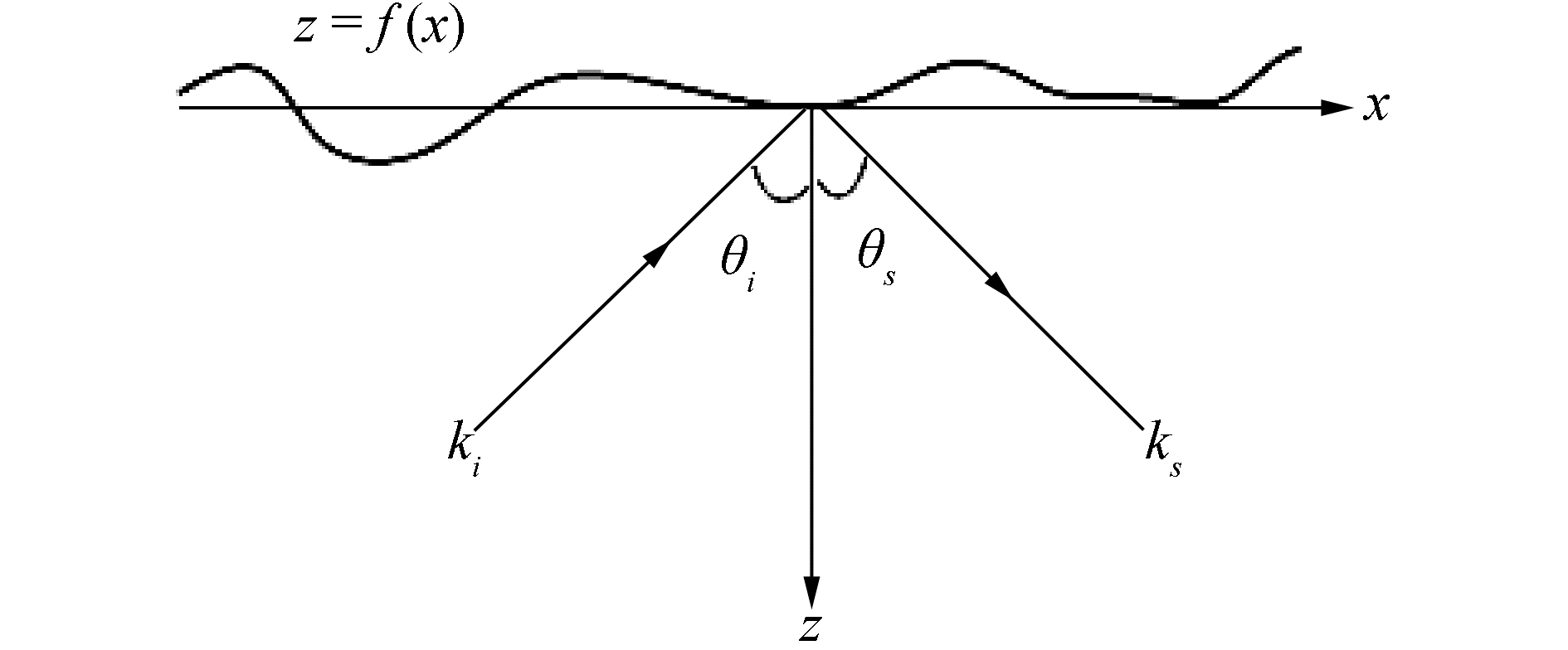Download: 图 1 一维粗糙海面散射示意 Fig. 1 Scattering sketch map for one-dimensional rough sea surface

 $\mathit{\boldsymbol{v}} = {\mathit{\boldsymbol{k}}_i}-{\mathit{\boldsymbol{k}}_s} = ({\mathit{\boldsymbol{v}}_x}, -{\mathit{\boldsymbol{v}}_z}), \;{\mathit{\boldsymbol{v}}_x} = {\mathit{\boldsymbol{k}}_{ix}}-{\mathit{\boldsymbol{k}}_{sx}}$
 ${\mathit{\boldsymbol{v}}_z} = {\mathit{\boldsymbol{\kappa }}_{iz}} + {\mathit{\boldsymbol{k}}_{sz}}, {\rm{ }}k{\beta _{1 + i}} = {[{k^2}-{({K_1} + {k_{ix}})^2}]^{1/2}}$

 ${\rm{Im}}[{\beta _{1 + i}}] > 0, {\rm{ }}k{\beta _{s - 1}} = [{k^2}-{({k_{sx}}-{K_1})^2}]$

 ${R_A}({k_{ix}}) \approx {\rm{exp}}\left[{-\frac{{{v_z}^2{h^2}}}{2}} \right][-1 + {\kappa _{iz}}\int {{\rm{d}}{K_1}W({K_1})g({K_1})}]$ (1)

1.2 粗糙海面下的声传播模型：Ramsurf

 $f({x_n}) = \frac{1}{L}\sum\limits_{{\rm{ }}j = -N/2 + 1}^{N/2} {F({k_j}){{\rm{e}}^{{\rm{i}}{k_j}{x_n}}}}$ (2)

 $\begin{array}{l} \;\;\;\;\;\;\;\;\;\;\;\;\;\;\;\;F({k_j}) = \frac{{2{\rm{ \mathsf{ π} }}}}{{\sqrt {2\Delta k} }}\sqrt {S({k_j})} \cdot\\ \left\{ \begin{array}{l} [N\left( {0, 1} \right) + iN\left( {0, 1} \right)], {\rm{ }}\;\;\;\;\;\;j = -N/2 + 1, \ldots, -1\\ N\left( {0, 1} \right), {\rm{ }}\;\;\;\;\;\;\;\;\;\;\;\;\;\;\;\;\;\;\;\;\;\;j = 0, N/2 \end{array} \right. \end{array}$ (3)

 $S\left( k \right) = \frac{{{h^2}l}}{{2\sqrt {\rm{ \mathsf{ π} }} }}{\rm{exp}}\left( {\frac{{-{k^2}{l^2}}}{4}} \right)$ (4)

2 数值仿真 2.1 粗糙海面声传播模型：Krakenc-KA和Krakenc-SSA 2.1.1 Krakenc-KA粗糙海面声场模型

Krakenc-KA声场模型有2种实现方法:1)直接设置ENV文件中的sigma参数(海面粗糙度参数)；2)首先通过KA方法计算出平均海面反射系数，再通过TRC(top reflection coefficient)文件输入到Krakenc中。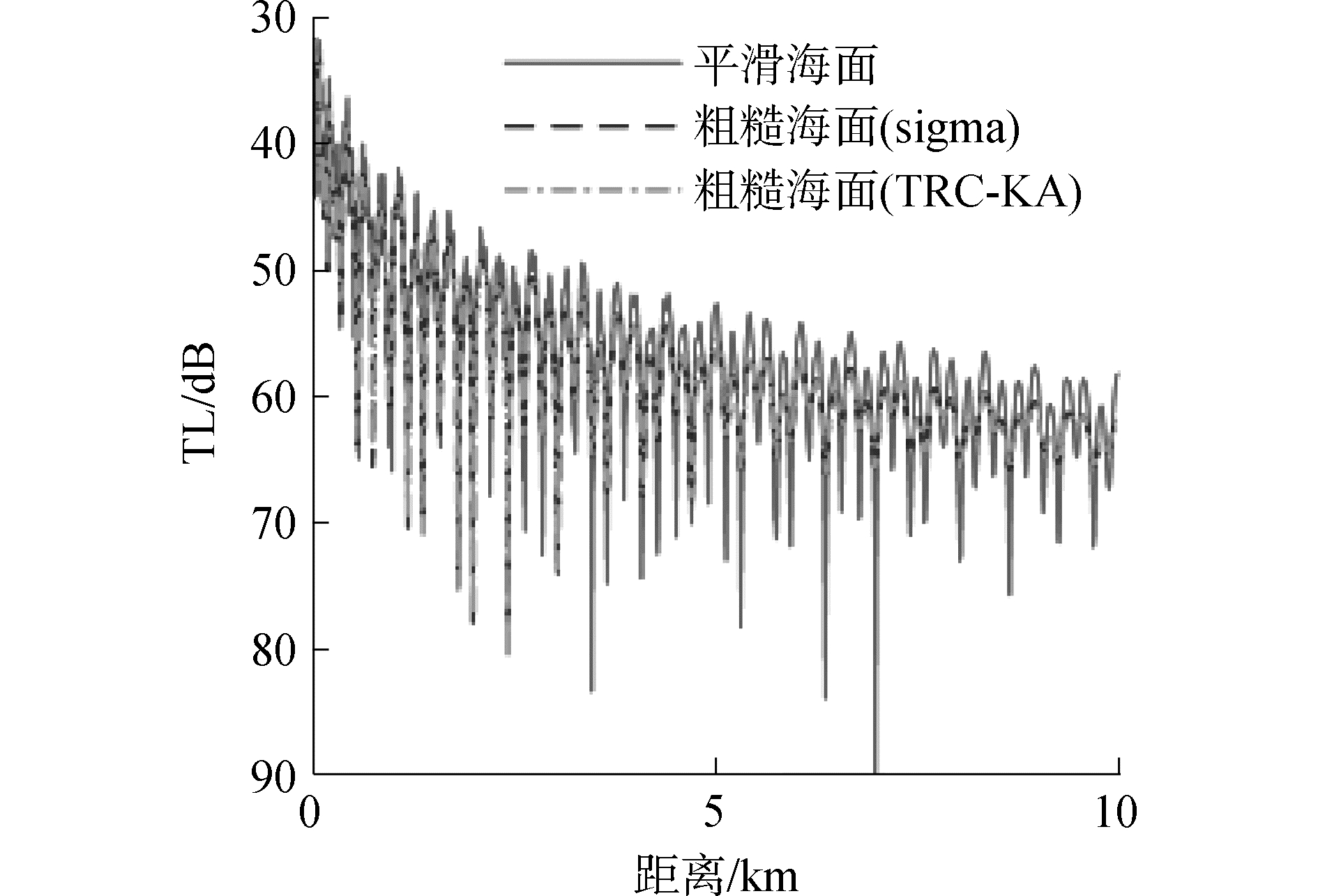Download: 图 2 Krakenc-KA粗糙海面声传播模型2种实现方法的比较 Fig. 2 Comparison of two realization methods for Krakenc-KA propagation model with rough sea surface
2.1.2 Krakenc-SSA粗糙海面声场模型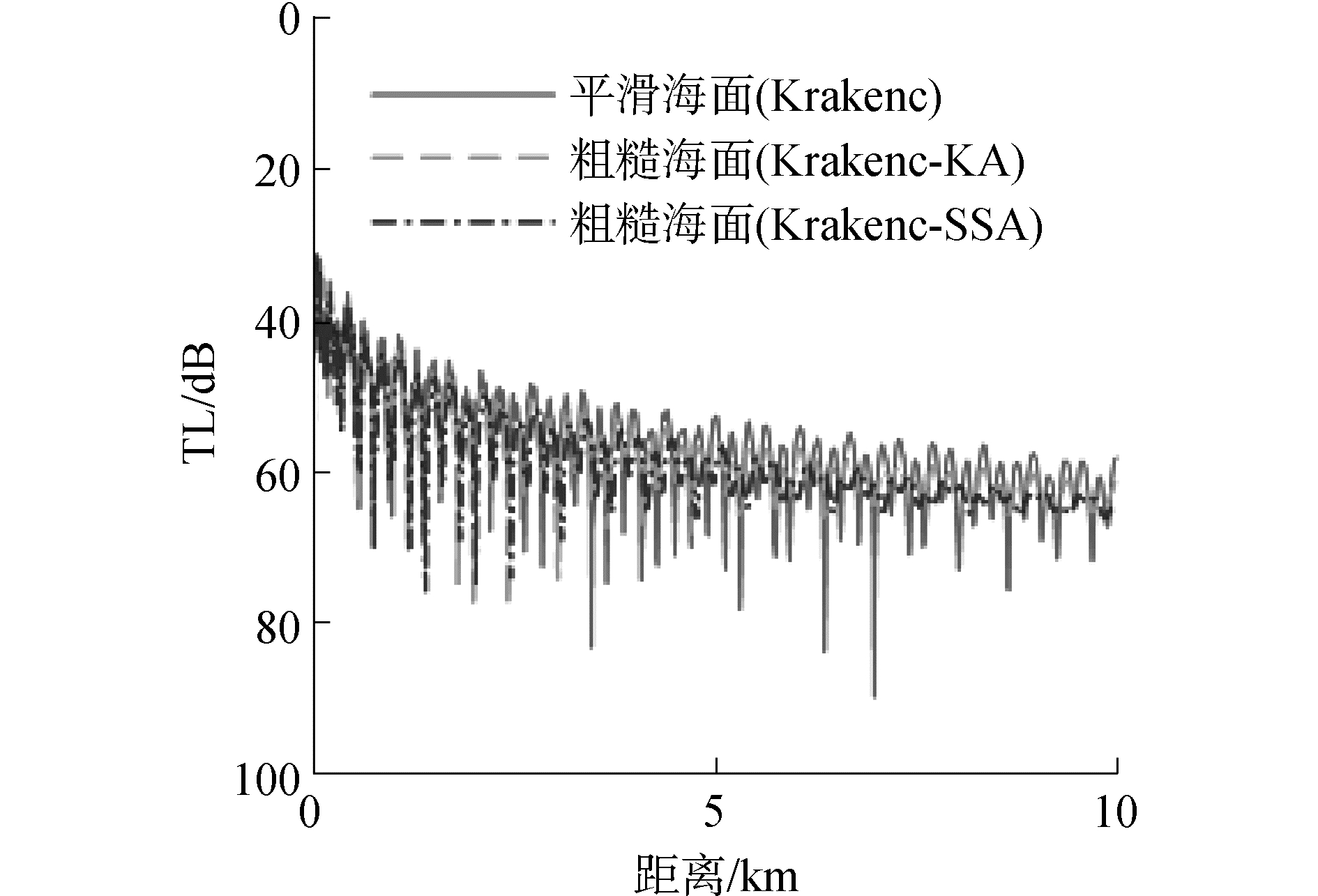Download: 图 3 不同散射方法计算海面平均反射系数时所得传播损失曲线的比较 Fig. 3 Comparison of transmission loss curves by different scattering method to calculate mean reflection coefficient

2.2 粗糙海面下的Krakenc模型与Ramsurf模型比较 2.2.1 平滑海面下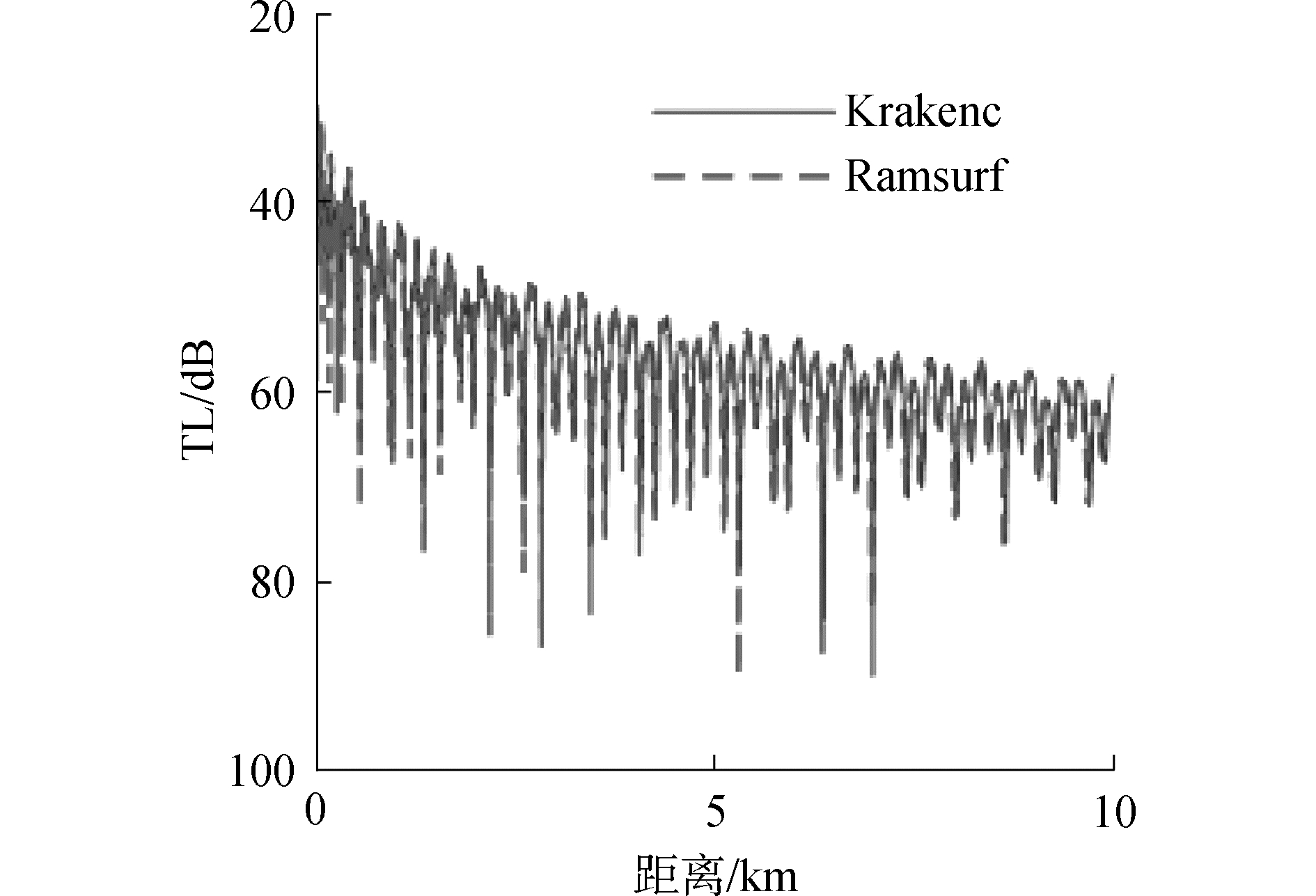Download: 图 4 平滑海面下Krakenc和Ramsurf的比较 Fig. 4 Comparison of transmission loss curves by Krakenc model and Ramsurf model with smooth sea surface
2.2.2 粗糙海面

Krakenc粗糙海面声传播模型是基于海面反射系数计算模型来计算平均海面反射系数，可根据实际需要选取多种不同的海面反射系数计算模型；而Ramsurf粗糙海面声传播模型是基于monte-carlo方法产生一维粗糙海面，可根据海区的具体情况选取多种不同的风浪谱模型。另外，Ramsurf可计算海底地形变化条件下的粗糙海面下的声场。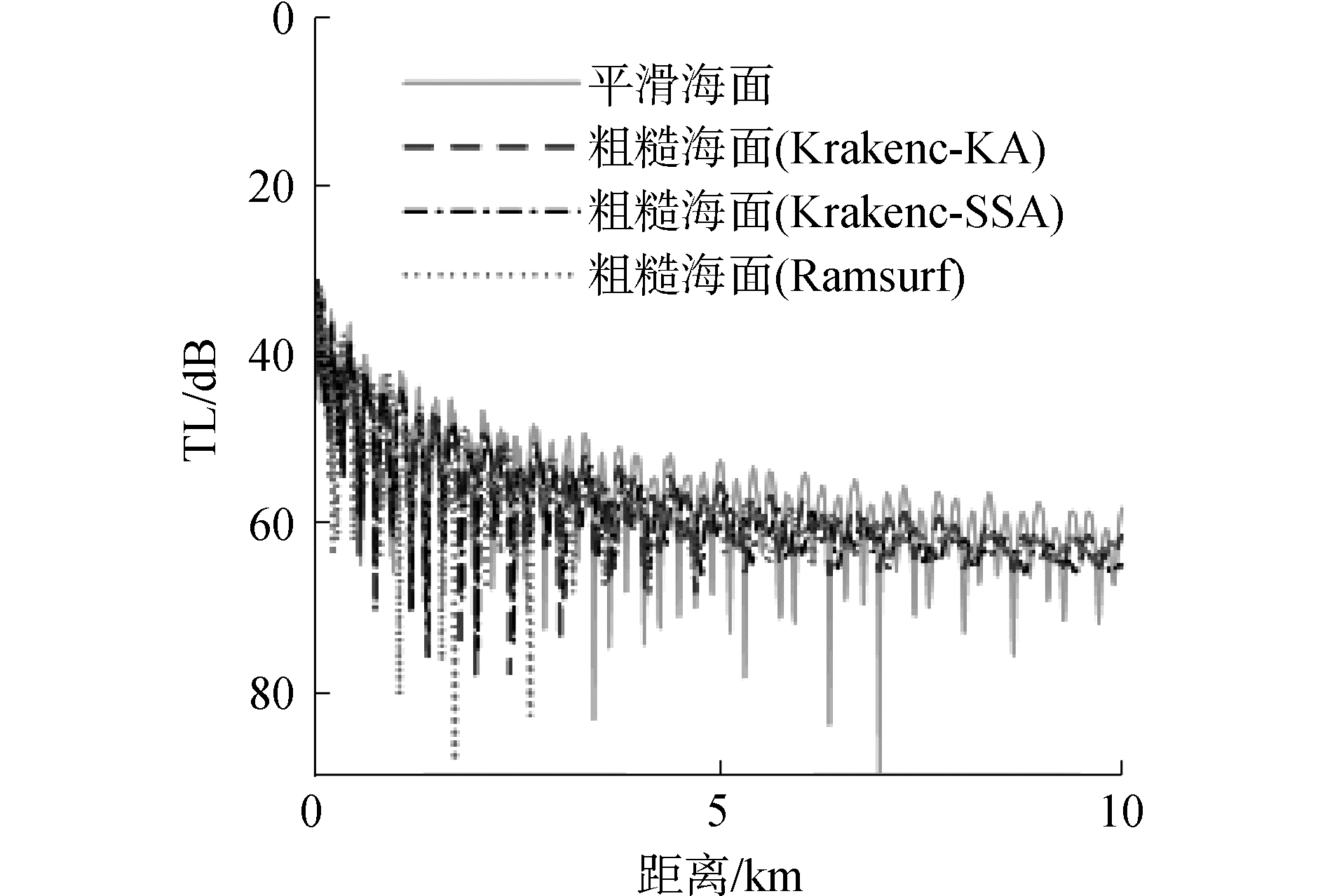Download: 图 5 粗糙海面下Krakenc-KA、Krakenc-SSA、和Ramsurf的比较 Fig. 5 Comparison of transmission loss curves by Krakenc-KA model, Krakenc-SSA model and Ramsurf model with rough sea surface

3 数据处理表 1 试验海区海底参数 Table 1 Seabed parameters of the sea area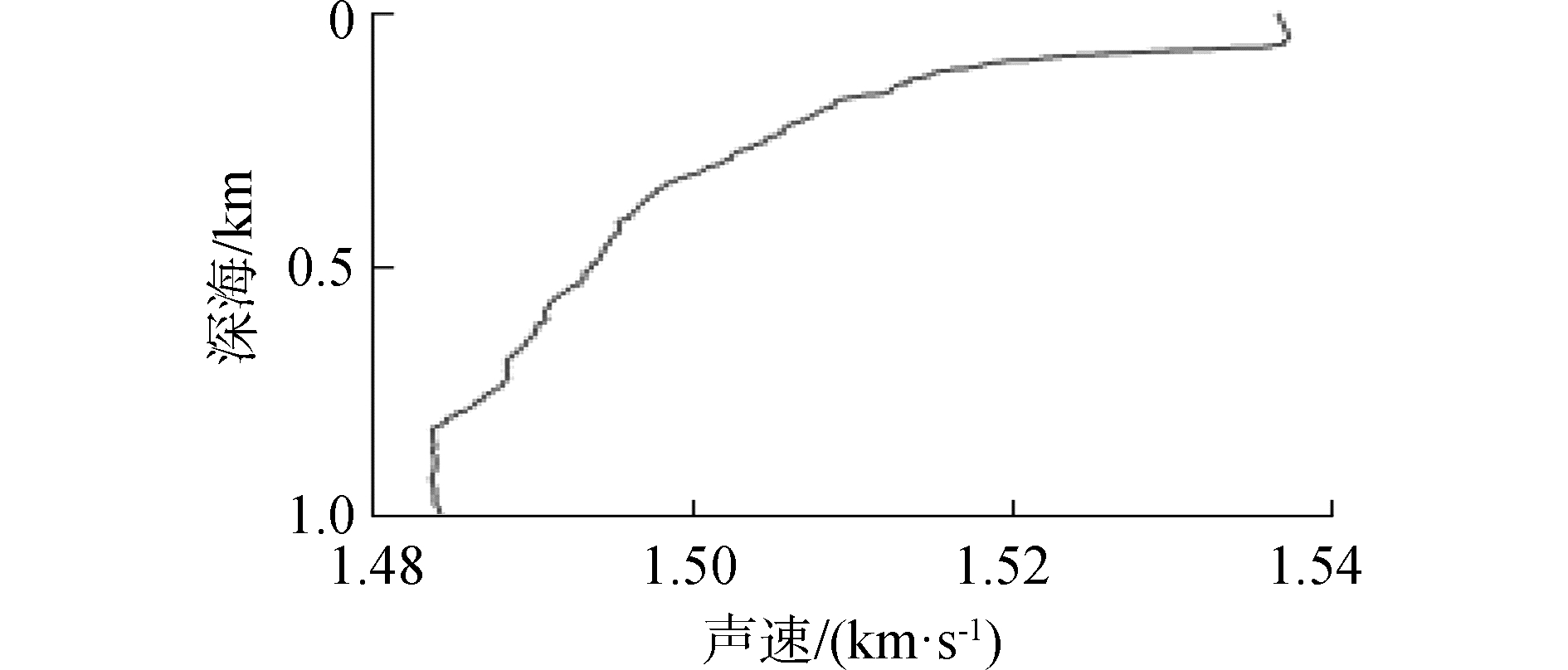Download: 图 6 试验地区声速剖面 Fig. 6 Sound speed profile of the sea area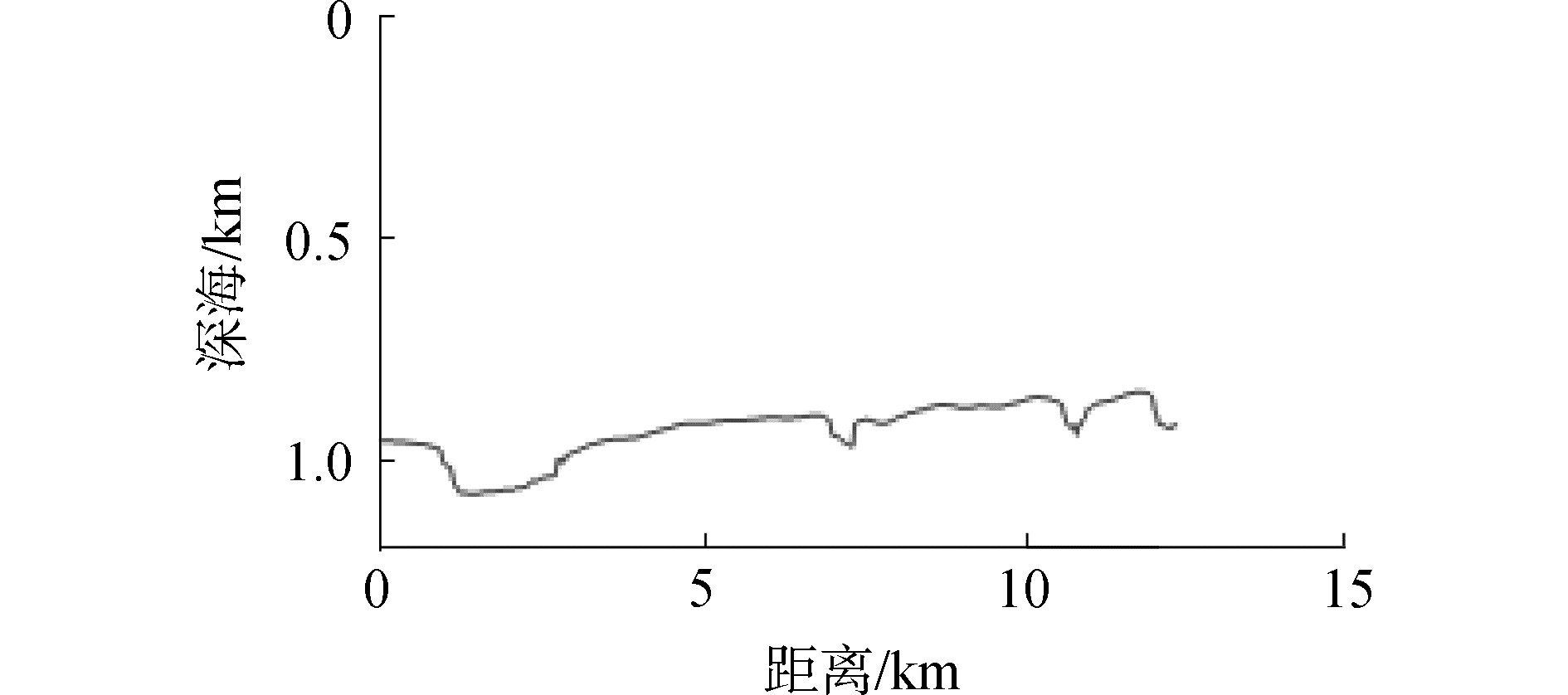Download: 图 7 试验地区海底地形 Fig. 7 Topography of the sea area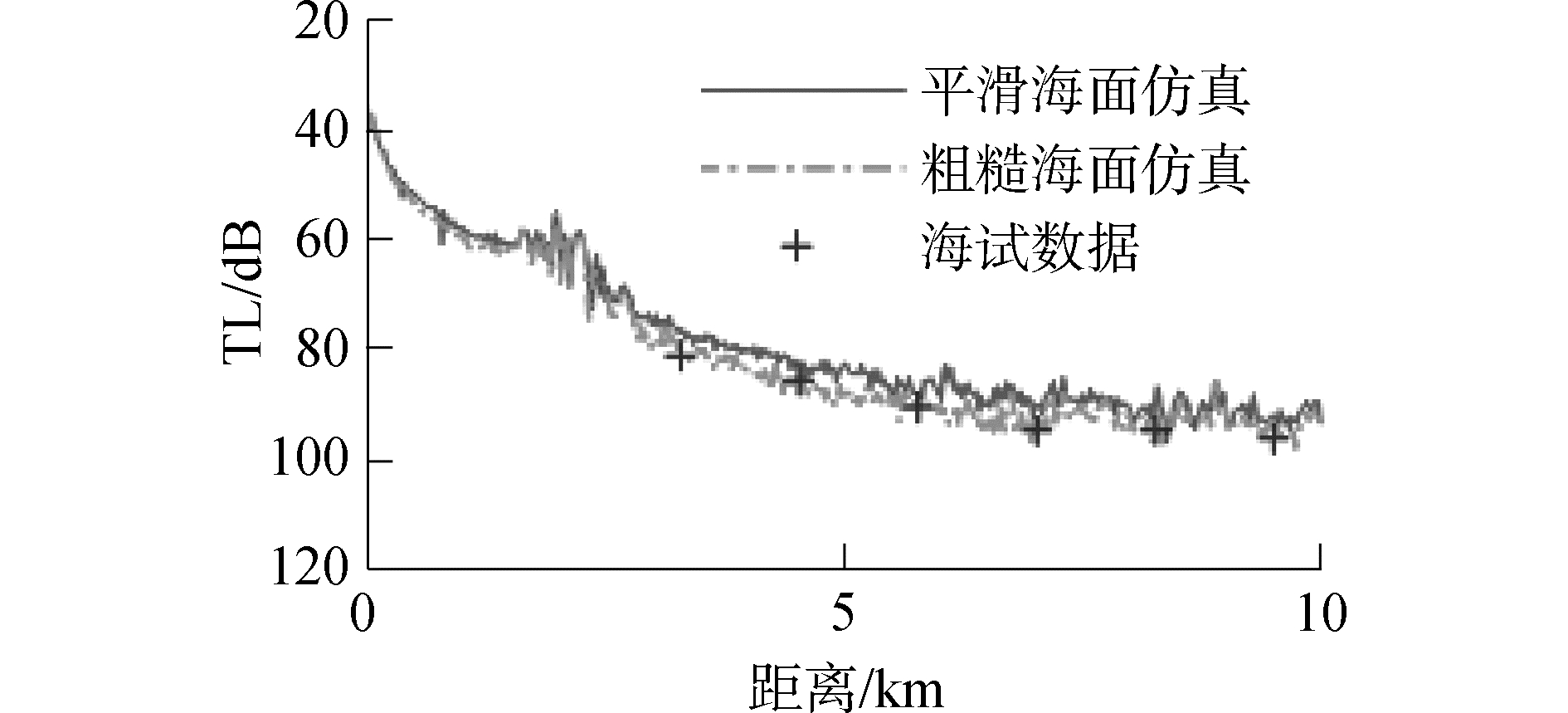Download: 图 8 Ramsurf传播损失仿真与海试处理结果比较(3 kHz) Fig. 8 Comparison of transmission loss curves by Ramsurf model and sea trial data with rough sea surface (3 kHz)
4 结论

1) KA方法计算的平均海面反射系数不改变声场的相位，而SSA方法计算的平均海面反射系数改变了声场的相位。因此，利用Krakenc-KA方法计算平均海面反射系数后得到的声场干涉峰谷的水平位置没有改变，而利用Krakenc-SSA方法计算平均海面反射系数后得到的声场改变了声场干涉峰谷的水平位置。

2) krakenc粗糙海面声传播模型是基于海面反射系数计算模型来计算平均海面反射系数，可根据实际需要选取多种不同的散射方法来计算平均海面反射系数；而Ramsurf粗糙海面声传播模型是基于monte-carlo方法产生一维粗糙海面，一般要进行40次以上的蒙特卡罗计算最后求得平均值，因此krakenc计算效率更高。

3) 通过实验数据验证Ramsurf是较为有效的粗糙海面下的声传播模型，并且可以计算粗糙海面下水平非均匀条件下的声场。

4) Krakenc-KA模型和Krakenc-SSA模型适用于水平均匀条件，文中的实验数据是海底地形变化条件下的数据，因此没有对其他两种模型进行验证。下一步的工作方向是获取更多的实验数据以开展对水平均匀条件下的Krakenc-KA模型和Krakenc-SSA模型的验证以及进一步的模型间的对比分析工作。

  QIN Jixing, LUO Wenyu, ZHANG Renhe, et al. Analysis and comparison between two coupled-mode methods for acoustic propagation in range-dependent waveguides[J]. Chinese journal of acoustics, 2014, 33(1): 1-20. (0)  LI Zhenglin, WANG Yaojun, MA Li, et al. Effects of sediment parameters on the low frequency acoustic wave propagation in shallow water[J]. Chinese journal of acoustics, 2000, 19(3): 221-229. (0)  MO Yaxiao, PIAO Shengchun, ZHANG Haigang, et al. An energy-conserving two-way coupled mode model for underwater acoustic propagation[J]. Chinese journal of acoustics, 2016, 35(2): 97-110. (0)  ZHANG Lingshan, PENG Zhaohui, WANG Guangxu, et al. Experimental analysis on air-to-water sound transmission loss in shallow water[J]. Chinese journal of acoustics, 2017, 36(2): 217-230. (0)  KIM I C, SUH K D. Effect of sea level rise and offshore wave height change on nearshore waves and coastal structures[J]. Journal of marine science and application, 2018(2): 192-207. (0)  BADIEV M, WAN L, LUO J. Shallow water modal evolution due to nonlinear internal waves[J]. Journal of marine science and application, 2017(3): 362-369. (0)  ZHOU Jixun, ZHANG Xuezhen, PENG Zhaohui, et al. Sea surface effect on shallow-water reverberation[J]. The journal of the acoustical society of America, 2007, 121(1): 98-107. DOI:10.1121/1.2400623 (0)  THORSOS E I. The validity of the Kirchhoff approximation for rough surface scattering using a Gaussian roughness spectrum[J]. The journal of the acoustical society of America, 1988, 83(1): 78-92. DOI:10.1121/1.396188 (0)  CHAPMAN D M F. An improved Kirchhoff formula for reflection loss at a rough ocean surface at low grazing angles[J]. The journal of the acoustical society of America, 1983, 73(2): 520-527. DOI:10.1121/1.388997 (0)  THORSOS E I. The validity of the Perturbation approximation for rough surface scattering using a Gaussian roughness spectrum[J]. J.Acoust.Soc.Am., 1989, 86(1): 261-277. DOI:10.1121/1.398342 (0)  SCHNEIDER H G. Surface loss, scattering, and reverberation with the split-step parabolic wave equation model[J]. The journal of the acoustical society of America, 1993, 93(2): 770-781. DOI:10.1121/1.405439 (0)  LEISHMAN T W, TICHY J. On the significance of reflection coefficients produced by active surfaces bounding one-dimensional sound fields[J]. The journal of the acoustical society of America, 2003, 113(3): 1475-1482. DOI:10.1121/1.1550924 (0)  WILLIAMS K L, THORSOS E I, ELAM W T. Examination of coherent surface reflection coefficient (CSRC) approximations in shallow water propagation[J]. The journal of the acoustical society of America, 2004, 116(4): 1975-1984. DOI:10.1121/1.1785617 (0)  THORSOS E I. Acoustic scattering from a "Pierson-Moskowitz" sea surface[J]. The journal of the acoustical society of America, 1990, 88(1): 335-349. DOI:10.1121/1.399909 (0)  JONES A D, MAGGI A L, CLARKE P A, et al. Analysis and simulation of an extended data set of waveforms received from small explosions in shallow oceans[C]//Proceedings of ACOUSTICS 2006. Christchurch, New Zealand, 2006. (0)  JONES A D, SENDT J, DUNCAN A J, et al. Modelling the acoustic reflection loss at the rough ocean surface[C]//Proceedings of ACOUSTICS 2009. Adelaide, Australia, 2009: 1-8. (0)  JONES A D, DUNCAN A J, MAGGI A L, et al. A detailed comparison between a small-slope model of acoustical scattering from a rough sea surface and stochastic modeling of the coherent surface loss[J]. IEEE journal of oceanic engineering, 2016, 41(3): 689-708. DOI:10.1109/JOE.2015.2464551 (0)  汪德昭, 尚尔昌.水声学[M].科学出版社, 2013 (0)